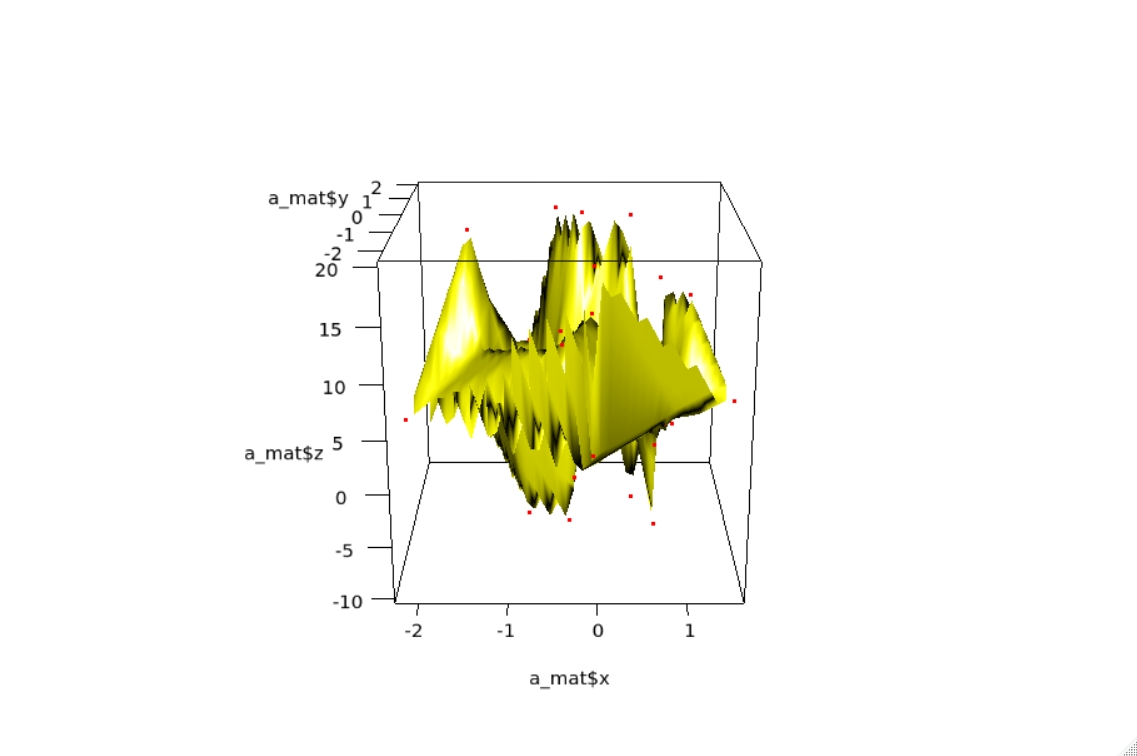# Create a 3D Surface Plot From (x,y,z) Coordinates

Jesse John May 31, 2022

Base R and several packages provide functions to plot a 3-dimensional surface over the horizontal plane. Most of them need a `z` value for every combination of `x` and `y` values as input.

In other words, they need an `x-y` grid and `z` values for each point on the grid.

When we have a data frame or matrix of `(x,y,z)` values (coordinates), we can plot the points, but not the surface.

We need to estimate `z` values for all combinations of `x` and `y` values using the given data and an appropriate interpolation function to plot the surface.

This article will show how to use the `akima` package to estimate the `z` values for a grid of `x-y` values. We will then plot the surface using the `rgl` package.

## the `interp()` Function of the `akima` Package in R

The `akima` package provides functions for interpolation of bivariate data.

The `interp()` function takes the three vectors `x`, `y` and `z` and returns a 40-by-40 grid of `x-y` values with a corresponding `z` value.

By default, a linear interpolation is produced.

## the `persp3d()` Function of the `rgl` Package in R

The `rgl` package creates 3D interactive graphics. We can zoom and rotate the plot using the mouse.

We will use the `persp3d()` function to plot the surface and the `points3d()` function to plot the original data.

In order to view the plots in R we need to set a particular option: `options(rgl.printRglwidget = TRUE)`.

## Demonstration of the Functions

We will go through the following steps.

• ##### Add the points using `points3d()`.

Example Code:

``````# INSTALL AND LOAD THE PACKAGES.
# Uncomment and run the concerned line to install the package.
#install.packages("akima")
library(akima)
#install.packages("rgl")
library(rgl)

# CREATE THE SAMPLE DATA.
set.seed(1)
x=rnorm(50)
set.seed(2)
y=rnorm(50)
z=c(rep(5,5),rep(-10,5), rep(3,15), rep(20,5), seq(5,15, length.out=10), rep(0,5), rep(3,5))
XYZ=data.frame(x,y,z)

# INTERPOLATE THE Z VALUES.
a_mat=interp(XYZ\$x, XYZ\$y, XYZ\$z)

# PLOT THE SURFACE AND THE POINTS.
options(rgl.printRglwidget = TRUE)
persp3d(a_mat\$x, a_mat\$y, a_mat\$z, col="yellow")
points3d(x,y,z, col="red")
``````

Output plot:

`rgl` produces an interactive 3D plot.Author: Jesse John

Jesse is passionate about data analysis and visualization. He uses the R statistical programming language for all aspects of his work.